# Bingo Probabilities for 90 balls

Updated:

## Introduction

These statistics come from the British Bingo game which can simulate the players

## How many times it takes a certain number of balls to get a “line” or a “house”, for a given number of players

### Graph of how many times it takes a certain number of balls to get a “line” or a “house”, for a given number of players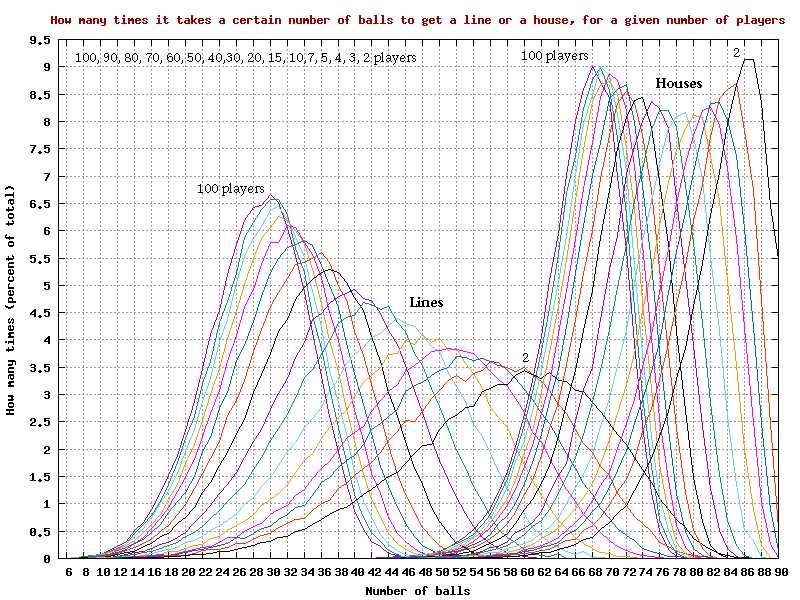Graph of how many times it takes a certain number of balls to get a 'line' or a 'house', for a given number of players

### Graphs of how many times it takes a certain number of balls to get a “line”, for a given number of players, in 3D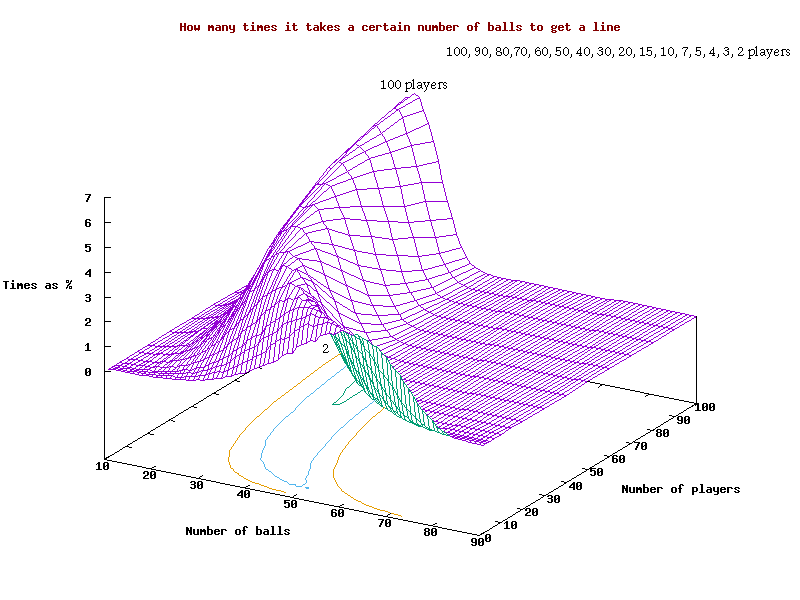Graph of how many times it takes a certain number of balls to get a 'line', for a given number of players, in 3D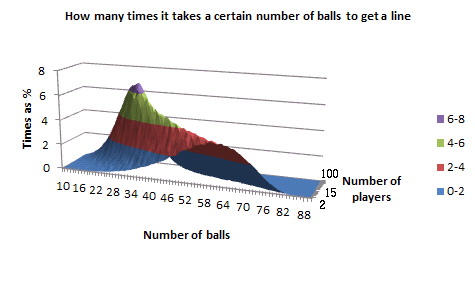Graph of how many times it takes a certain number of balls to get a 'line', for a given number of players, in 3D, as a surface chart in Excel

### Graphs of how many times it takes a certain number of balls to get a “house”, for a given number of players, in 3D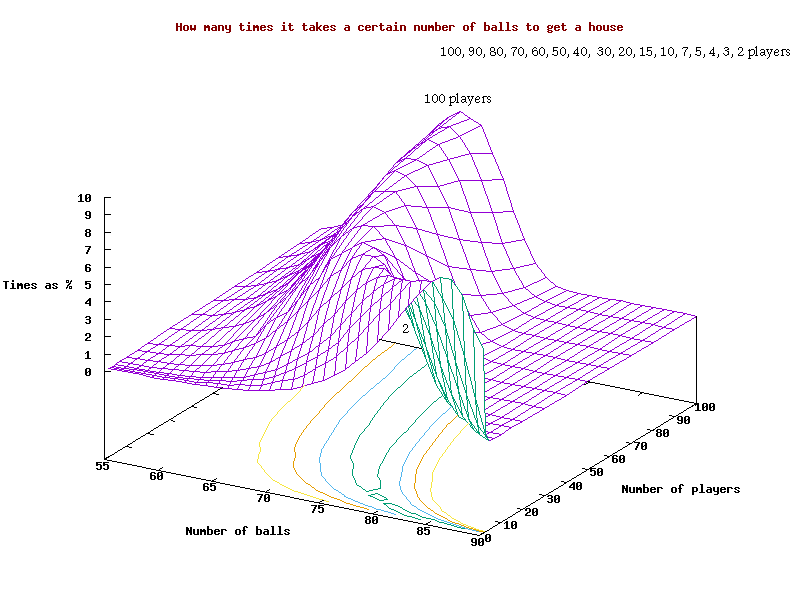Graph of how many times it takes a certain number of balls to get a 'house', for a given number of players, in 3D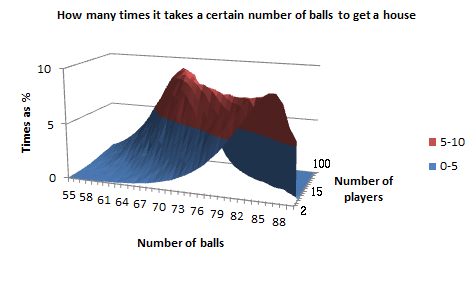Graph of how many times it takes a certain number of balls to get a 'house', for a given number of players, in 3D, as a surface chart in Excel

## Average number of balls to get a “line” and “house” for a range of number of players

### Graph of the average number of balls to get a “line” or “house” for 2 to 50 players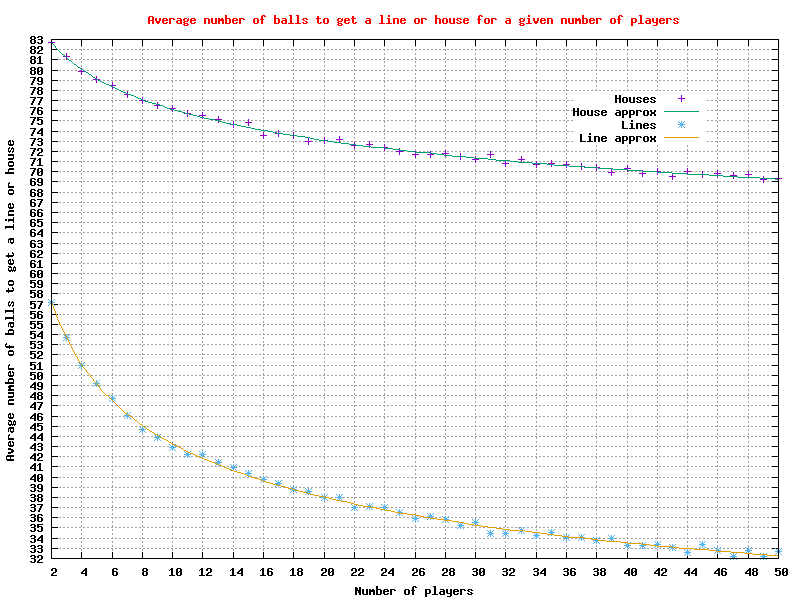Graph of the average number of balls to get a 'line' or 'house' for 2 to 50 players

### Graph of the average number of balls to get a “line” or “house” for 2 to 200 playersGraph of the average number of balls to get a 'line' or 'house' for 2 to 200 players

### Graph of the average number of balls to get a “line” or “house” for 2 to 300,000 players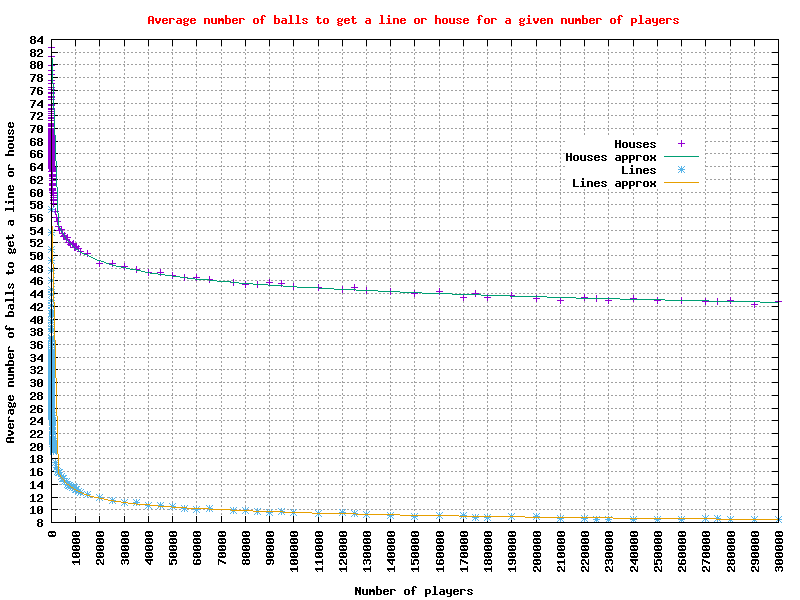Graph of the average number of balls to get a 'line' or 'house' for 2 to 300,000 players